Next: 3.1.5 The Constant Quasi-Fermi Up: 3.1 Sets of Partial Previous: 3.1.3 The Hydrodynamic Transport

## 3.1.4 The Lattice Heat Flow Equation

To account for self-heating effects in semiconductor devices, the lattice heat flow equation has to be solved which reads
 div SL = H -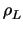. cL .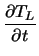(3.15) SL = -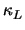. grad TL (3.16)

with SL being the lattice heat flow density. The coefficients of this equation are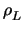, cL, and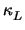, which denote the materials mass density, specific heat, and thermal conductivity, respectively. H is the generated local heat density and is modeled in dependence of the transport model. In the drift-diffusion case H equals the Joule heat,

 H = grad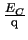-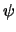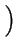. Jn + grad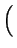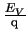-. Jp   , (3.17)

whereas in the hydrodynamic case the relaxation terms are used 

 H =.n .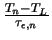+ p .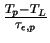. (3.18)Next: 3.1.5 The Constant Quasi-Fermi Up: 3.1 Sets of Partial Previous: 3.1.3 The Hydrodynamic Transport
Tibor Grasser
1999-05-31